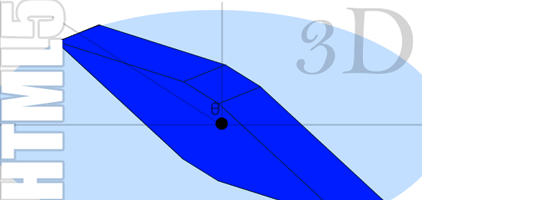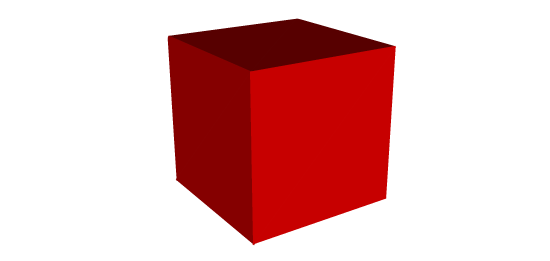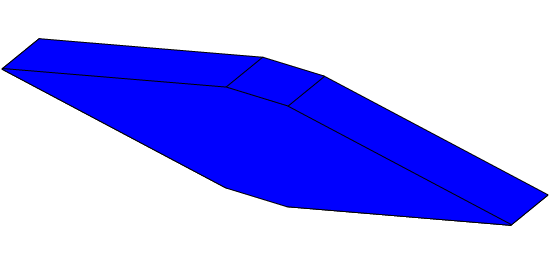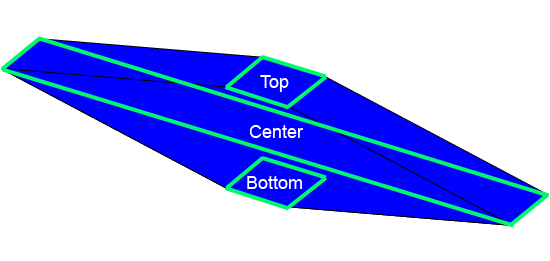# How to Create an HTML5 3D EngineYou’re probably aware by now that HTML5 is game-changing. In particular, with the HTML5 canvas, there’s been an outpouring of HTML5 3D JavaScript engines such as three.js released to enhance the rudimentary 2D HTML5 API. After all, 3D graphics is a lot cooler than 2D graphics, right?

It’s probably fair to say that if you were to create a full-blown 3D engine–complete with three dimensional matrix transformations, point objects, plane objects, shading, ray tracing, and other spatial computations–it would be quite difficult. But what if we wanted to create an optimized, barebones 3D JavaScript engine that supports translations and rotations? How hard would it be?

What if I tell you that we can create our own simple 3D JavaScript engine with just 40 lines of code? This guide will provide a simple and straightforward JavaScript engine that enables HTML5 3D renderings that can be used in web animation, games, and so forth.

### Understanding 3D Projections

Before creating our own 3D engine, we should first understand how the illusion of 3D space is created on a 2D screen.

These illusions are called 3D projections. 3D projections map points onto a two-dimensional plane. In our case, the three-dimensional points define an object we wish to render, and the two-dimensional plane is the computer screen. As you can see in the following image, we can create a three-dimensional projection of a cube on a two-dimensional plane by drawing three irregular quadrilaterals – the top, left, and front quadrilaterals.### Plane Projections with Ellipses

Unfortunately, calculating three-dimensional projections can be quite complex to code. How can we simplify it? Imagine looking down at a rotating rectangular plane.

Its four corners would lie on the outline of a perfect circle, whose radius is equal to half the distance from opposite corners of the rectangle. Now imagine if we were to tilt the plane in 3D space. What happens?

This imaginary circle suddenly becomes an ellipse, whose height is less than its width. This means that we could create a simple 3D projection by creating planes whose corners reside along the edges of an ellipse. If we are defining the rotation of the plane with an angle, `theta`, the 3D projection calculations suddenly become quite simple!

Any point on our three-dimensional plane can be defined with the following two equations which describe a point along the edges of an ellipse:

`x = A * cos(theta) y = B * sin(theta)`

where `A` is half of the ellipse width, and `B` is half of the ellipse height.

### A Simple 3D Engine

If our engine simply creates planes in 3D space, we can build multiple cross-sectional planes that frame a 3D object that we wish to render.

#### Sample 3D Object Rendering#### Cross-Sectional Planes DiagramView demo This particular 3D shape was created by generating three cross-sectional planes – the top, center, and bottom plane.

These imaginary planes provide us with all of the points we need to render the 3D object using the HTML5 Canvas API. By creating a straightforward function that can generate, translate, and rotate these cross-sectional planes, we have effectively created a simple 3D engine. Here is the code for our HTML5 3D engine:

`// This simple 3D engine was provided by www.Html5CanvasTutorials.com // for the purpose of creating 3D HTML5 renderings function Plane(centerX,centerY, planeLength, planeWidth, planeTilt, planeTheta) { this.centerX = centerX; this.centerY = centerY; this.planeLength = planeLength; this.planeTheta = planeTheta; var lastPerspectiveX = null; var lastPerspectiveX2 = null; var planeNextCornerAngle = 2*Math.asin(planeWidth/planeLength); this.rotate = function(newTheta) { planeTheta = newTheta - planeNextCornerAngle/2; } this.translate = function(newCenterX, newCenterY) { centerX = newCenterX; centerY = newCenterY; } this.generate = function() { var ovalLength = planeLength; var ovalWidth = ovalLength * planeTilt; var perspectiveX = (ovalLength / 2) * Math.cos(planeTheta); var perspectiveY = (ovalWidth / 2) * Math.sin(planeTheta);             var perspectiveX2 = (ovalLength / 2) * Math.cos(planeTheta + planeNextCornerAngle); var perspectiveY2 = (ovalWidth / 2) * Math.sin(planeTheta + planeNextCornerAngle); this.topLeftX = (perspectiveX *1) + centerX; this.topLeftY = (perspectiveY * -1) + centerY; this.bottomRightX = (perspectiveX*-1) + centerX; this.bottomRightY = (perspectiveY*1) + centerY this.topRightX = (perspectiveX2 *1) + centerX; this.topRightY = (perspectiveY2 *-1) + centerY; this.bottomLeftX = (perspectiveX2 *-1) + centerX; this.bottomLeftY = (perspectiveY2 *1) + centerY; } }`

This simple 3D engine is completely free to use and modify so long as you keep the commented credits in your code.

Enjoy!